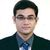# App Development

Announcements
Coming Aug. 9: New Simplified Authoring for Qlik Sense SaaS – For Details, CLICK HERE
cancel
Showing results for
Did you mean:Creator

## Summing up Like terms in a field and finding individual avg based on the like terms

Hello all,

I have a similar table in my data load:

 Product Number MPG A B 111aaaa A5 21 2.2 222bbb B6 53 6 3c3c3c A5 56 31 As34s43 9T 54 12 ADSWRW22 B6 432 12 FWE544SD 9T 214 56 ADS540-FD A5 32 5

So what i want to do is collect like terms in the MPG column and (A-B)/B for each distinct MPG. After finding the Change, i would also like to find individual avg of EACH MPG. so basically divide the change by the number of times an MPG occurs in the original table.

Resulting Table: (NOTE: Change and AVG(Change) columns don't have to be separated as long as the avg is found)

 MPG Change Individual AVG(Change) A5 (21-2.2)/2.2 + (56-31)/31 ((21-2.2)/2.2 + (56-31)/31 + (32-5)/5)/3 B6 (53-6)/6 + (432-12)/12 ((53-6)/6 + (432-12)/12)/2 9T (54-12)/12 + (214-56)/56 ((54-12)/12 + (214-56)/56)/2

You help is appreciated.

Thank you.

Labels (3)

• ### LikeTerms

1 Solution

Accepted SolutionsMVP

Hi,

Sum(Aggr((Sum(A)-Sum(B))/Sum(B),[Product Number],MPG))

and the dimension is MPG

Regards,
Kaushik
7 RepliesMVP

Hi,

Sum(Aggr((Sum(A)-Sum(B))/Sum(B),[Product Number],MPG))

and the dimension is MPG

Regards,
KaushikCreator
Author

Hi,

is it possible to divide it by the number of MPG occurrences after?

So for example, all the values will be divided by 2 in the table above since each MPG appears twice. Basically like finding AVG after the sum.

Thank youMVP

HI,

Can you explain with some example and expected output.

Regards,
KaushikCreator
Author

Hi,

For sure, i can expand on my question. So, the resulting table would be something similar to the following table:

 MPG Change A5 ((21-2.2)/2.2 + (56-31)/31)/2 B6 ((53-6)/6 + (432-12)/12)/2 9T ((54-12)/12 + (214-56)/56)/2

so basically, after the expression you wrote above, i would like to divide it by the number of time a MPG occurs. So, in the table above, A5 appears twice and so does the other two MPGs.Creator
Author

Hi,

the expression you gave in your reply, sums everything up for one MPG value. it doesnt put them in their respective place.

FIGURE1:my chart expression is as follows:

Sum(Aggr((Sum(FSIML2NP)-Sum("C L2 NP"))/Sum("C L2 NP"),[oM_PN],"F MPG"))

does the product number in your expression have to be uniqe? what is the use of?

Thank youMVP

Where did "Code Value" come from? It's not in the original question.

If the data is as shown in your original post and the dimension is MPG, I think the correct expression is:

Sum((A-B)/B) / Count([Product Number])

-RobCreator
Author

Hi Rob,

"Code Value" is MPG in the original post. Also, im not sure why would you divide it by Count[Product Number]. The reason i have /2 in one of my replies is because each MPG appears twice in the original table. In my actual dataset, one MPG value could appear 200 times.

your expression would just divide it by the total numbers of product numbers. my problem requires diving it by the number of time EACH MPG occurs in a table.